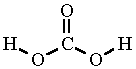# Why does carbonate have a valence of two?

Nov 12, 2016

The valency of carbonate is two because it can combine with two hydrogen atoms.

#### Explanation:

Valency is the number of univalent atoms (e.g., $\text{H}$ or $\text{Cl}$ atoms) that can combine with an atom or an ion.

The structure of carbonate isEach $\text{O}$ atom has a negative charge, so it can combine with an $\text{H"^"+}$ ion to form carbonic acid.Since carbonate can combine with two $\text{H}$ atoms, its valency is two.